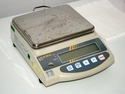## Algebraic Reasoning

Type of Unit: Concept

Prior Knowledge

Students should be able to:

• Add, subtract, multiply, and divide rational numbers.
• Evaluate expressions for a value of a variable.
• Use the distributive property to generate equivalent expressions including combining like terms.
• Understand solving an equation or inequality as a process of answering a question: which values from a specified set, if any, make the equation or inequality true?
• Write and solve equations of the form x+p=q and px=q for cases in which p, q, and x are non-negative rational numbers.
• Understand and graph solutions to inequalities x<c or x>c.
• Use equations, tables, and graphs to represent the relationship between two variables.
• Relate fractions, decimals, and percents.
• Solve percent problems included those involving percent of increase or percent of decrease.

# Lesson Flow

This unit covers all of the Common Core State Standards for Expressions and Equations in Grade 7. Students extend what they learned in Grade 6 about evaluating expressions and using properties to write equivalent expressions. They write, evaluate, and simplify expressions that now contain both positive and negative rational numbers. They write algebraic expressions for problem situations and discuss how different equivalent expressions can be used to represent different ways of solving the same problem. They make connections between various forms of rational numbers. Students apply what they learned in Grade 6 about solving equations such as x+2=6 or 3x=12 to solving equations such as 3x+6=12 and 3(x−2)=12. Students solve these equations using formal algebraic methods. The numbers in these equations can now be rational numbers. They use estimation and mental math to estimate solutions. They learn how solving linear inequalities differs from solving linear equations and then they solve and graph linear inequalities such as −3x+4<12. Students use inequalities to solve real-world problems, solving the problem first by arithmetic and then by writing and solving an inequality. They see that the solution of the algebraic inequality may differ from the solution to the problem.

Subject:
Mathematics, Algebra
Material Type:
Unit of Study
Level:
Middle School
7
Provider:
Pearson
Tags:
Integers, Inequalities, Area, Perimeter, 7th Grade Mathematics, Expresssions, Distributive Property, Concepts, Negative Numbers, Assessment, Operations, Estimation, Algebraic Expressions, Variables, Graphing, Measurement, Problem-Solving, Expressions, Equations, Triangles
Unit 5 Algebraic Reasoning# Education Standards (28)

•••••••••Lesson 9

Peer Review
•••••••